# Development of an underwater robotic arm using multibody dynamics approach

## S. Fernando1, M. Perera2

1School of Civil and Mechanical Engineering, Curtin University, Perth, WA 6845, Australia

2Department of Mechanical Engineering, SLIIT Campus, Malabe, Sri Lanka

1Corresponding author

Vibroengineering PROCEDIA, Vol. 40, 2022, p. 120-125. https://doi.org/10.21595/vp.2021.22301
Received 15 November 2021; received in revised form 1 December 2021; accepted 10 December 2021; published 5 February 2022

54th International Conference on Vibroengineering, Shanghai, China, February 5, 2022

Copyright © 2022 S. Fernando, et al. This is an open access article distributed under the Creative Commons Attribution License, which permits unrestricted use, distribution, and reproduction in any medium, provided the original work is properly cited.
Views 130
CrossRef Citations 0
Abstract.

Underwater robotic arms are important devices that enables workers to carry out tasks remotely from a safe distance reducing or eliminating the risks that are involved with the task. The primary objective of the robotic manipulator is to perform maintenance and cleaning activities of the hull of a ship. However, the control of these devices underwater is quite complicated due to the numerous factors that make these systems unstable and non-linear. The aim of this study is to develop a multibody dynamic robotic manipulator model, integrated with a control strategy to optimize and obtain stable kinematics solutions. The hydrodynamic forces are integrated to the manipulator model considering buoyancy forces and surface drag forces. A basic algorithm is used to generate the joint angles using 7 geometrical parameters. The control of the manipulator was done to simply follow any path that represents the given coordinates. The P, I and D parameters are tuned individually to optimize the kinematic solution of the manipulator. 3-DOF articulated manipulator is the commonly used manipulator configuration. However, a 6-DOF manipulator configuration was selected in this study to allow for change in orientation using wrist motions.

Highlights
• Trajectory planning to optimize travelling path for 6 DOF robotic manipulator
• Inverse kinematics using dimensional approach
• 2DOF stabilization of base connected ROV

Keywords: robotic manipulator, inverse kinematics, underwater robotics, multibody dynamics, 6DOF manipulator, manipulator control.

#### 1. Introduction

Ships or vessels that are submerged partially or completely underwater are constantly in contact with various living organisms that are inhabited in these bodies . These organisms tend to get contaminated the surfaces of submerged bodies resulting in degrading of the hull and even causing significant amounts of drag opposing the motion of the vessel.

Throughout the history, engineers have incorporated various techniques to clean the surface, by manual and automated methods. These cleaning methods are mainly divided as, Dry-dock cleaning and underwater cleaning . Underwater cleaning was used to be done by professional divers going to the bottom of the vessel and manually cleaning the hull which was however mostly ineffective and unsafe. More recent advancements in technology enabled complete remotely operated cleaning of the hull surface of the ships. When it comes to operations such as welding and painting the accuracy of the movement is very important which is very difficult to obtain due to both inertia and hydrodynamic forces. The scope of this investigation is to develop a 6 DOF underwater robotic manipulator which will move a desired cleaning system (Laser Cleaning or pressure cleaning) around a 3D space providing the necessary accuracy.

When designing a manipulator, DH parameters is an important data set that is required to analyze the kinematics of the model . However,  shows a method that can be used to find the kinematic solutions of a model of up to 6 DOF by using 7 basic parameters which can be easily derived from the manipulator dimensions. This study has used the above method to solve the kinematic solutions to the model. In addition to that, for underwater systems, fluid dynamic and buoyancy forces must be considered simultaneously when controlling the manipulator . The positioning of the end effector while the base is connected to a floating body has a strong inertia and hydrodynamic coupling. The controller should take all these into consideration simultaneously for precise operation . In this study a virtual model has been built in multibody dynamics environment where all these physical phenomena can be connected together such that the actual control strategy can be fully developed, and necessary gains can be selected. Furthermore, authors are expected to extend this work by incorporating the component flexibility and tribological aspects of the contact conjunctions of the robot arms. Such an extension will only be possible in the multibody dynamics environment which uses the Lagrange methods to formulate the equations . Through this the accuracy of the end effecter will further be improved.

Fig. 1. 7 Parameters used for solving kinematics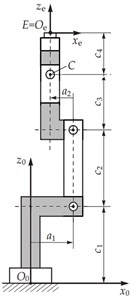a) Side view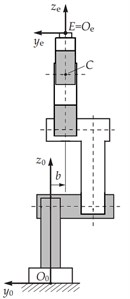b) Back view

#### 2. Mathematical model of the 6DOF manipulator

The initial simulations for the robotic manipulator designs were done using MATLAB Simulink multibody-dynamics environment by importing the CAD models built from solid works. However, the models were later transferred to ADAMS software since it was simpler to visualize the mechanics of the model. Then, for the implementation of the control system, MATLAB-ADAMS co-simulation was used.

After the open loop kinematics analysis, it was later decided that the use of a 6 DOF manipulator with wrist joints would be much beneficial due to its ability to change the orientation of the end effector frame . The three degrees of freedom manipulator lacked the ability to change the orientation of the end-effector frame. This issue was solved by upgrading to a higher degree of freedom system.

Table 1. Parameters of the 6-DOF manipulator that were used in the solution of the inverse kinematics

 Parameter a1 a2 b c1 c2 c3 c4 Value (mm) 0 0 0 110 300 135 135

The definition of above parameters in Table 1. that are used in solving the inverse kinematic solutions for the 6 revolute joints representing the 6-DOF can be found in the Fig. 1. 7 Parameters used for solving kinematics.

The fluid dynamic forces were introduced to the manipulator and the inverse kinematics for the 6-DOF system was developed after carefully reviewing the literature of the kinematic analysis for the manipulator.

The fluid dynamic forces considered in this study are the buoyancy forces and the profile drag forces that are acting on the model. The formula for the buoyancy forces acting on the centre of buoyancy of each link can be expressed as shown below:

(1)
${F}_{B}={\rho }_{fluid}×{V}_{eff}×g,$

where ${\rho }_{fluid}$ is the density of the fluid, ${V}_{eff}$ is the effective volume of fluid that is displaced due to the body and g is the gravitational acceleration.

The profile drag component of the fluid dynamic forces can be expressed as a function of the square of the velocity $\left({v}^{2}\right)$ of the body, the drag coefficient ${C}_{d}$, the density of the fluid and the effective area that $A$. The formula for the profile drag force acting on the centroid of the links due to the motion of the manipulator can be expressed as shown below:

(2)
${F}_{PD}=0.5{C}_{d}×{\rho }_{fluid}×A{v}^{2}.$

Fig. 2. a) Rotated view and b) Side view of the robotic manipulator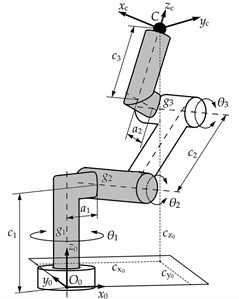a) Rotated view of manipulator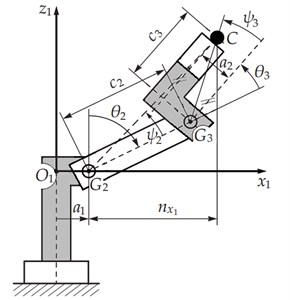b) Side view of manipulator

The kinematic solutions of the of the system were implemented based on the method introduced in  by using the dimensional parameters of the designed robotic manipulator given in Table 1. The expressions for the forward kinematics of the model can be derived as:

(3)
${c}_{x0}={c}_{x1}\mathrm{cos}{\theta }_{1}-{c}_{y1}\mathrm{sin}{\theta }_{1},$
(4)
${c}_{y0}={c}_{x1}\mathrm{sin}{\theta }_{1}+{c}_{y1}\mathrm{cos}{\theta }_{1},$
(5)
${c}_{z0}={c}_{z1}+{c}_{1},$

where, ${c}_{x1}$, ${c}_{y1}$, ${c}_{z1}$ are the coordinates of the tip of the end effector from the side view shown in Fig. 2(b) and ${c}_{x0}$, ${c}_{y0}$, ${c}_{z0}$ are the coordinates of the final position of the end effector relative to the given joint angles.

The first two of the multiple solutions related to the first three joint angles can be derived using the inverse kinematic equations which can be expressed as shown below:

(6)
${\theta }_{1}={\mathrm{tan}}^{-1}\frac{{c}_{y0}}{{c}_{x0}}±{\mathrm{tan}}^{-1}\frac{b}{{n}_{x1}+a},$
(7)
${\theta }_{2}=±{\mathrm{cos}}^{-1}\frac{{s}_{1}^{2}+{c}_{2}^{2}-{k}^{2}}{2×{s}_{2}{c}_{2}}+{\mathrm{tan}}^{-1}\frac{{n}_{x1}}{{c}_{z0}+{c}_{1}},$
(8)
${\theta }_{3}=±{\mathrm{cos}}^{-1}\frac{{s}_{1}^{2}-{c}_{2}^{2}-{k}^{2}}{2×{s}_{2}{c}_{2}}-{\mathrm{tan}}^{-1}\frac{{a}_{2}}{{c}_{3}},$

where, ${\theta }_{1}$, ${\theta }_{2}$, ${\theta }_{3}$ are the first two solutions of the positioning joints, ${n}_{x1}$ is the distance between ${G}_{2}$ axis and $C$ and:

(9)
${s}_{1}={n}_{x1}^{2}+{\left({c}_{z0}-{c}_{1}\right)}^{2},$
(10)
${s}_{2}={\left({n}_{x1}+2{a}_{1}\right)}^{2}+{\left({c}_{z0}-{c}_{1}\right)}^{2},$
(11)
$k=\sqrt{{a}_{2}^{2}+{c}_{3}^{2}}.$

#### 3. Results and discussion

Th results given in this paper are based on the 6-DOF manipulator model and the square shaped desired trajectory 1-4 given in Fig. 3.

Fig. 3. Labelled diagram of the 6-DOF manipulator Adams model with the trajectory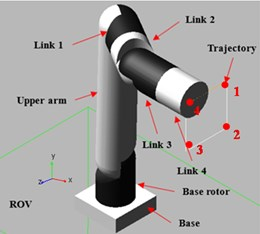After the implementation of the inverse kinematics of the manipulator and the control strategy using PID controllers, it was identified that the velocity profile of the joint motions had to be controlled to achieve a smooth motion with minimum amount of peaking of the joint torques. For this, a trajectory with polynomial velocity profile was developed using Robotic Operating Systems (ROS) toolbox in Matlab Simulink. The resulting polynomial velocity profiles are shown in Fig. 4 and Fig. 5.

Fig. 4. Polynomial velocity trajectory of the Base joint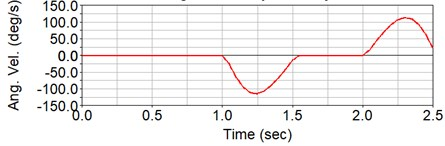Fig. 5. Angular velocities around the $z$ axis of the six individual joints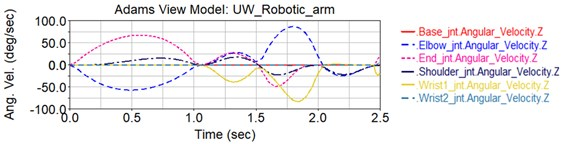In order to establish the hydrodynamic environment, the buoyancy forces and the drag forces were introduced to the multibody model using the Adams software.

The buoyancy forces acting on each link of the manipulator arm are always constant since the manipulator is fully submerged inside the water body during the operation. Therefore, the buoyancy force acting on each link can be calculated using the effective volume of the link ${V}_{eff}$ and Eq. (1).

The drag forces applied on the upper arm around the global $y$ axis can be graphically shown as below in Fig. 6.

The graphical representation of the drag force acting on the links 1 and 2 around the global $z$ axis and the global $y$ axis due to the motion can be shown as in Fig. 7 and Fig. 8.

Fig. 6. The drag force acting on the upper arm due to the motion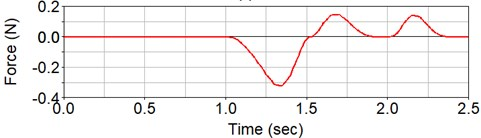Fig. 7. Drag force acting on the Links 1, 2 around the global $z$ axis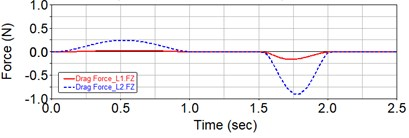Fig. 8. Drag force acting on the Links 1, 2 around the global $y$ axis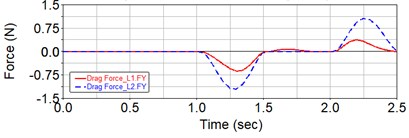When the manipulator is in motion, there will be forces induced as a result of the inertial forces and the hydrodynamic forces available in the system. The resultant of these forces will act on the ROV at the base of the manipulator where it is mounted to the ROV. They also create a torque around the global $y$ axis of the manipulator. The resultant torque will act on the ROV at the centre of mass.

The vertical force that will be created at the base of the 6-DOF manipulator can be shown as in Fig. 9 and the torque that will be created at the centre of mass of the ROV can be shown as in Fig. 10.

Fig. 9. The total vertical force acting on the ROV due to the inertial forces and the hydrodynamic forces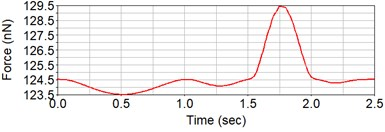Fig. 10. The total torque acting on the ROV due to the inertial forces and the hydrodynamic forces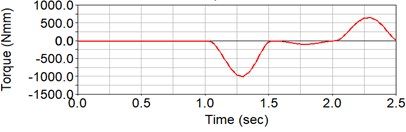To achieve optimum control of the manipulator, the PID controllers of each individual 6 degrees of freedom were tuned separately .

After tuning the PIDs, the resulting torque at the base joint was observed as shown below in Fig. 11.

Fig. 11. Torque needed for the motion at the base joint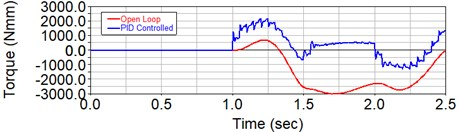Fig. 12. Deviation of actual end-effector $x$ coordinate from the desired $y$-$z$ plane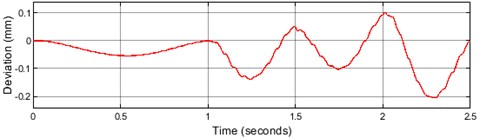The trajectory given is on a on the $z$-$y$ plane. This makes the displacement in the $x$ direction constant. The deviation of the end-effector $x$-coordinate with reference to the desired $x$-coordinate after the tuning of the PIDs is shown by Fig. 12.

#### 4. Conclusions

The main objective of the project was to develop and implement the control strategy of a robotic manipulator that is supposed to be used in the shipping industry for maintenance purposes. The initial design of the robotic manipulator yielded certain limitations. These limitations were overcome by improving the initial design of 3 degrees of freedom to a 6 degree of freedom system. The trajectory planning was done to make the end-effector follow the path that represent the coordinates that were input to the model, and the final results of the joint motions were analyzed. The forces and torques applied at each joint to achieve the desired motion were analyzed and controlled using Feedback PID Control. Further improvements can be done to optimize the control of the manipulator to improve sensitivity of the trajectory of the end effector.

#### Acknowledgements

The author wishes to thank Aaqil Zackariya of University of SLIIT’s Mechanical Engineering Department for their guidance, advice and assistance in making this project a success.

1. C. Song and W. Cui, “Review of underwater ship hull cleaning technologies,” Journal of Marine Science and Application, Vol. 19, No. 3, pp. 415–429, Sep. 2020, https://doi.org/10.1007/s11804-020-00157-z [Publisher]
2. M. W. Spong, S. Hutchinson, and M. Vidyasagar, “Robot modeling and control [book review],” IEEE Control Systems, Vol. 26, No. 6, pp. 113–115, Dec. 2006, https://doi.org/10.1109/mcs.2006.252815 [Publisher]
3. Mathias Brandstötter, A. Angerer, and M. Hofbaur, “An analytical solution of the inverse kinematics problem of industrial serial manipulators with an ortho-parallel basis and a spherical wrist,” in Proceedings of the Austrian Robotics Workshop, pp. 7–11, 2014. [Search CrossRef]
4. T. J. Tarn, G. A. Shoults, and S. P. Yang, “A dynamic model of an underwater vehicle with a robotic manipulator using Kane’s method,” Autonomous Robots, Vol. 3, No. 2-3, pp. 269–283, 1996, https://doi.org/10.1007/bf00141159 [Publisher]
5. E. Kelasidi and K. Y. Pettersen, “Modeling of underwater snake robots,” in Encyclopedia of Robotics, Berlin, Heidelberg: Springer Berlin Heidelberg, 2018, pp. 1–9, https://doi.org/10.1007/978-3-642-41610-1_50-1 [Publisher]
6. A. D. Analysis and M. Systems, Chapter 1 ADAMS / Solver and MSS. pp. 1–138. [Search CrossRef]
7. G. Ellis, “Four Types of Controllers,” Control System Design Guide, pp. 97–119, 2012, https://doi.org/10.1016/b978-0-12-385920-4.00006-0 [Publisher]